Maths-
General
Easy

Question

# If the relation between subnormal 'SN' and subtangent 'ST' at any point on the curve by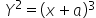is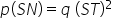then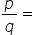##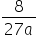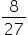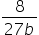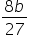Hint:

## The correct answer is:### The given curve is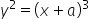.The relation between subtangent "ST" and subnormal is "SN" is p(SN) = q(ST)2.We have to find the ratio of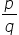.Subtangent: It is the projection of tangent on x-axis from the x intercept to point of tangency.Subnormal: It is the projection of normal on x-axis from the x intercept to point of tangency.The formula of subtangent is ST=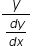The formula of subnormal is SN =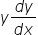we will differentiate the curve w.r.t xNow,  p(SN) = q(ST)2For such questions, we should know the formulas of subnormal and subtangent.#### With Turito Foundation.#### Get an Expert Advice From Turito.